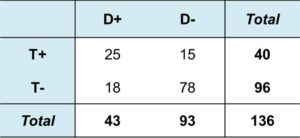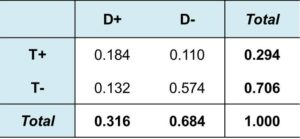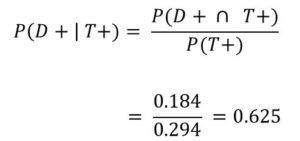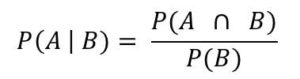# Conditional Probability – Basics

I assume you have a basic knowledge of Probability. For example, if there a 3 red ball and 2 blue balls in a bag and someone picks on ball at random. What is the probability that it is a red ball? Or what is the probability of getting a 3 if a fair six-sided die is rolled? I’m sure you know it.

But just in case you forgot, for the former,  it’s 3/5 and for the later, it is 1/6.

You may also want to to read Decision Theory.

Now, conditional probability is also quite easy to understand. It simply tried to determine the probability of an event given a previous event.

We would use an example to explain Conditional Probability.

#### Medical Diagnosis Example

A medical diagnosis is carried out on patients with a heart disease called digitalis intoxication.  To carry out this diagnoses, doctors measure the concentration of digitalis in the patient’s blood. (high concentration of digitalis does not automatically mean that this disease is present, but it make it more likely).

So 136 patient’s were diagnosed and the results are tabulated below.

• T+  = High concentration
• T –  = low concentration
• D+ = disease present
• D-  = disease absentLet’s now understand what is happening here.

The first figure 25,  shows that 25 patient out of 136 has high concentration and also has the disease. This is 0.184

Also, 15 patients out of 136 has high concentration but does not have the disease.

Now before continue, let’s convert the table to frequencies, so we can apply it to larger population. We simply divide everything by 136. The resulting table is shown below.

The figures can now represent probabilities.From the table:

P(T+) = 0.294

P(D+) = 0.316

We can now ask: ‘if a doctor know that there was high concentration(that is the test was positive T+), what is the probability of the disease being present?’  This is conditional probability.

Looking only at the first row, we can see that out of the 40 patients who shows high concentration, 25 of them has the disease.

Let’s denote  the probability that a patient has the disease given that the test is positive by P(D+ | T+).

This means conditional probability of D+ given T+.

In this case

P(D+ | T+) = 25/40 = 0.625

In the same way, we can calculate this probability asSo this is what conditional probability is all about.

But now we can also think of unconditional probability.

From our calculation we can see that  the unconditional probability of D+ is 0.316 while the conditional probability of T+  given D+ is 0.625. This is almost twice the previous.

This means that given that the test is positive (T+) makes the disease twice more likely to be present.

Now what if the test is negative (T-)?

Again, we can also find:

P(D- | T-) = 0.574/0.706 = 0.813

We can then check the unconditional probability of D- which is 0.684 from the table.

#### False Positive and False Negative

We can then find probability of false positive. That is probability that the patient has the disease given a negative test result (T-). We can represent this as:

P(D- | T+) = 0.375

Finally, the probability of False negative is given by:

P(D+ | T-) = 0.1875

#### Formal Definition of Conditional Probability

Now that you clearly understand conditional probability, let’s now give a formal definition.

If A and B are two events with P(B) ≠ 0. Then the conditional probability of A given  B is given by:The idea behind this is that if we are given that an even B occurred, then the relevant sample space becomes B rather than the original sample size. And the condition probability is the probability measured over B.

It the medical diagnosis example we calculate  P(D+ | T+) over the 40 patients who have high concentration (T+)

You may also want to to read Decision Theory.#### kindsonthegenius

Kindson Munonye is currently completing his doctoral program in Software Engineering in Budapest University of Technology and Economics

View all posts by kindsonthegenius →